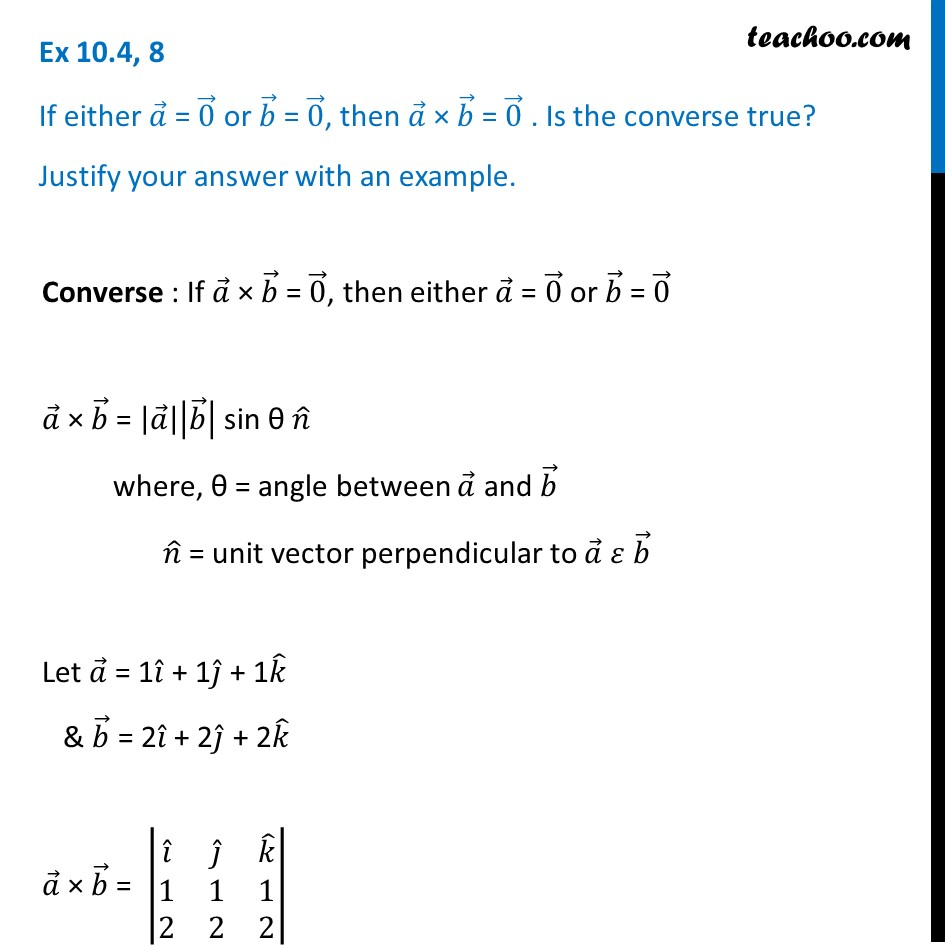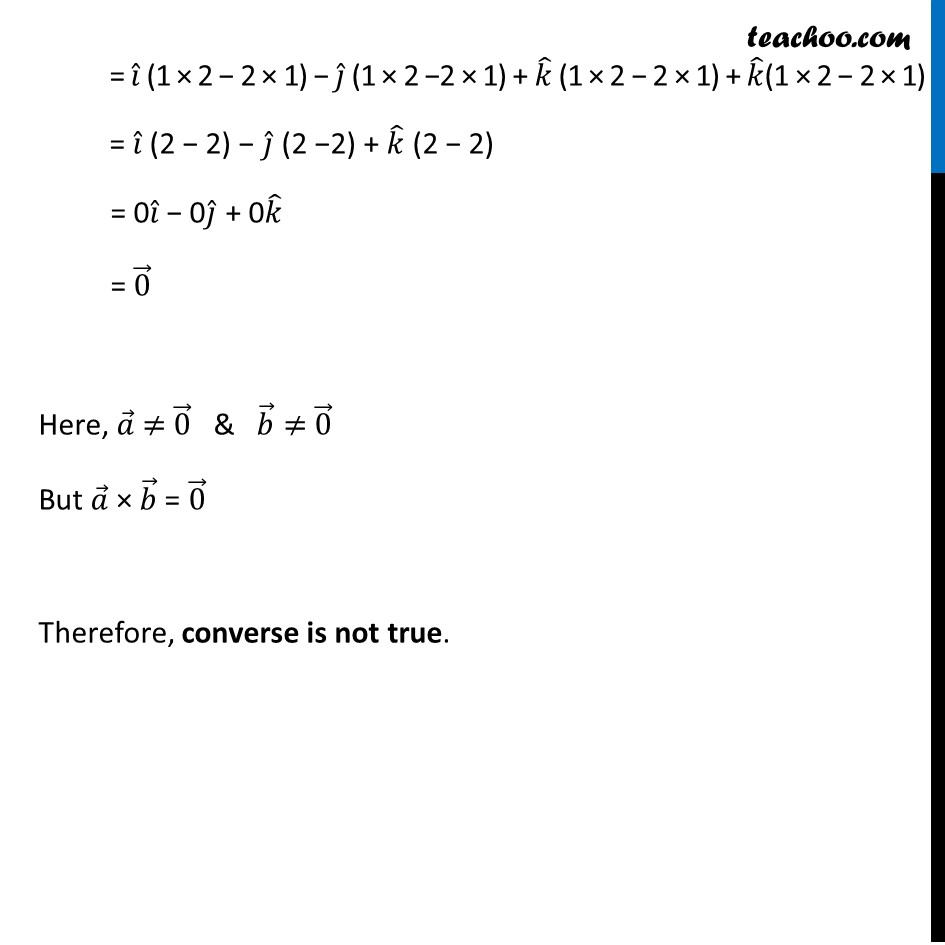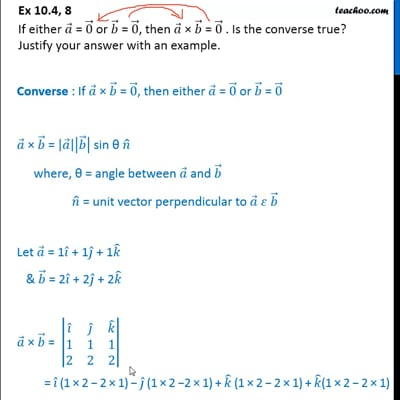Ex 10.4

Chapter 10 Class 12 Vector Algebra
Serial order wiseThis video is only available for Teachoo black users

Get live Maths 1-on-1 Classs - Class 6 to 12

### Transcript

Ex 10.4, 8 If either 𝑎 ⃗ = 0 ⃗ or 𝑏 ⃗ = 0 ⃗, then 𝑎 ⃗ × 𝑏 ⃗ = 0 ⃗ . Is the converse true? Justify your answer with an example. Converse : If 𝑎 ⃗ × 𝑏 ⃗ = 0 ⃗, then either 𝑎 ⃗ = 0 ⃗ or 𝑏 ⃗ = 0 ⃗ 𝑎 ⃗ × 𝑏 ⃗ = |𝑎 ⃗ ||𝑏 ⃗ | sin θ 𝑛 ̂ where, θ = angle between 𝑎 ⃗ and 𝑏 ⃗ 𝑛 ̂ = unit vector perpendicular to 𝑎 ⃗ 𝜀 𝑏 ⃗ Let 𝑎 ⃗ = 1𝑖 ̂ + 1𝑗 ̂ + 1𝑘 ̂ & 𝑏 ⃗ = 2𝑖 ̂ + 2𝑗 ̂ + 2𝑘 ̂ 𝑎 ⃗ × 𝑏 ⃗ = |■8(𝑖 ̂&𝑗 ̂&𝑘 ̂@1&1&[email protected]&2&2)| = 𝑖 ̂ (1 × 2 − 2 × 1) − 𝑗 ̂ (1 × 2 −2 × 1) + 𝑘 ̂ (1 × 2 − 2 × 1) + 𝑘 ̂(1 × 2 − 2 × 1) = 𝑖 ̂ (2 − 2) − 𝑗 ̂ (2 −2) + 𝑘 ̂ (2 − 2) = 0𝑖 ̂ − 0𝑗 ̂ + 0𝑘 ̂ = 0 ⃗ Here, 𝑎 ⃗ ≠ 0 ⃗ & 𝑏 ⃗≠ 0 ⃗ But 𝑎 ⃗ × 𝑏 ⃗ = 0 ⃗ Therefore, converse is not true.